## 输入

Scaner scaner = new Scaner (System.in);
//输入数字
int number = scaner.nextInt();
//输入字符串
String string = scaner.next();//不接受下面的符号，也就是输入下面的符号，不会结束当前的输入
String string = scaner.nextLine();//接收回车、换行、TAB
next()nextLine()输入区别

nextInt()、nextFloat()之类函数与next()特性一致。

• next() 必须先进行有效输入（不包括结束符号的字符）才能结束输入（否则一直是等待输入的状态），然后再使用结束符号结束当前输入（回车、换行、TAB、空格的任一种）
• nextLine()：就没有上面约束了，能接收任何字符，结束符号只有回车一个，所以可以输入含有空格的字符串。

• next() 接收回车，结束输入后，\n 不会消失（要么被下一个next()过滤，要么被下一个nextLine()接收
• nextLine() 接收回车，结束输入后，会去除掉\n，所以下一个nextLine()可以正常接收输入，而不会接收到回车

• 有效输入，next() 必须要接收到有效输入，才能结束输入。nextLine()可以直接接收回车结束字符（实际上接收为空""，因为会自动去除回车）。
• 结束符号种类不同
• 对结束符号回车的处理不同

### 格式化输入

OJ有有一种输入是A B，就是两个输入，中间有一个空格。

### 多组数据输入，不确定组数，以EOF结束。

while(sc.haveNext()){
//
}

## 输出

// 第一种
DecimalFormat df = new DecimalFormat("#0.00");
float data;
data = sc.nextFloat();
System.out.println(df.format(data));

// 第二种,模仿c语言的输出方式，但是不会换行
System.out.printf("%.2f\n", data);// 保留两位小数
System.out.printf("%5d",n);//域宽为5

% - 0 m.n [类型符号]

①%：表示格式说明的起始符号，不可缺少。
②-：有-表示左对齐输出，如省略表示右对齐输出。
③0：有0表示指定空位填0,如省略表示指定空位不填。
④m.n：m指域宽，即对应的输出项在输出设备上所占的字符数。（如果输出位数少于m位，左端默认补空格，字符数是指整个实数的位置，包括小数点）n指精度。用于说明输出的实型数的小数位数。为指定n时，隐含的精度为n=6位。
⑤[类型符号] d表示整数  f表示浮点数

// 第三种
System.out.println(String.format("%.2f", data));

## 基本数据类型

int、float、double、char、String

String不算基本数据类型，应该是类了。

int 4字节（32bit） -2^31 ~ 2^31 - 1
short 2字节（16bit） -2^15 ~ 2^15 - 1
long 8字节（64bit）-2^63 ~ 2^63 - 1
float 4字节（32bit）2^-149 ~ 2^128 - 1
double 8字节（64bit）2^-1074 ~ 2^1024 - 1
char 1字节

### 常用的方法

//比较大小
//按字典顺序比较两个字符串。
a.compareTo(b);//如果a>b 返回大于0的数

//类型转换
int Interger.parseInt(String s);
Interger Interger.valueOf(String/int s);

//判断是否是数字
Character.isDigit(String s);

### 进制转化

//其他进制转十进制
Integer.valueOf("[数字字符串]",[进制数]).toString()

//十进制转二进制
Integer.toBinaryString(int i)

//十进制转八进制
Integer.toOctalString(int i)

//十进制转十六进制
Integer.toHexString(int i) 

### 字符串

#### 取子串

//substring 方法用于提取字符串stringObject中介于两个指定下标[start,stop)之间的字符。stop的位置不会被取到，不写stop，默认到字符串末尾
testString.substring(start,stop);

//没有length，一直取到末尾
stringObject.substr(start [, length ]);


#### 子串查找

string.indexof(String s);//返回第一次出现的指定子字符串在此字符串中的索引。
string.indexof(String s, int startIndex);//从指定的索引处开始，返回第一次出现的指定子字符串在此字符串中的索引。

lastIndexOf(String str) //返回在此字符串中最右边出现的指定子字符串的索引。
lastIndexOf(String str, int startIndex) //从指定的索引处开始向后搜索，返回在此字符串中最后一次出现的指定子字符串的索引。
?

string.contains(String s);

#### 字符查找

string.charAt(index)

#### 字符数组转换

//char数组转String
char data[] = {'s', 'g', 'k'};
String str = new String(data);
str = String.valueOf(data);

#### 字符串构造

//字符串构造
StringBuilder s = new StringBuilder();
StringBuilder s = new StringBuilder(String s);//直接把字符串作为参数
s.append(String s);
s.reverse() //反转序列
s.delete(int start,int end)//移除指定范围字符
s.insert(int offset,  i)//在指定位置插入字符或者字符串，可以说包含了append的功能
s.replace(int start, int end, String str)//用新的字符串替换指定序号范围的子字符串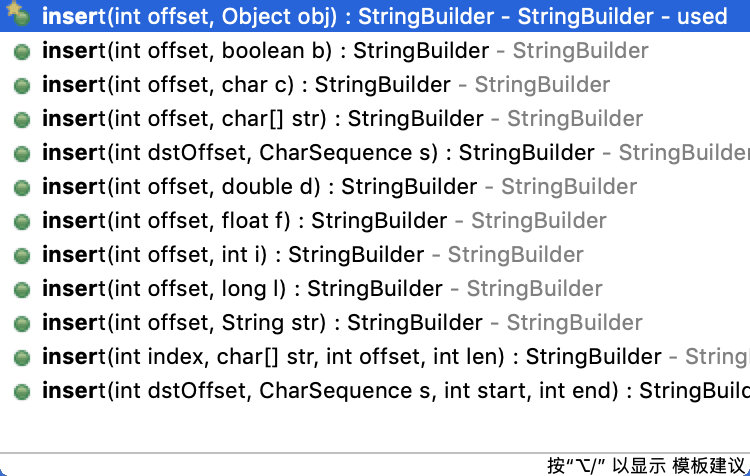#### 替换

• String replace方法不会修改自身字符串内容，只是返回一个新的字符串
• StringBuilder 是按范围替换一段新的字符串，String是按字符或者按正则匹配，替换所有匹配上的地方
string.replace(char a,char b);//把所有的a字符替换成b字符
string.replaceAll(regex, String replace);//根据正则表达式替换

## 集合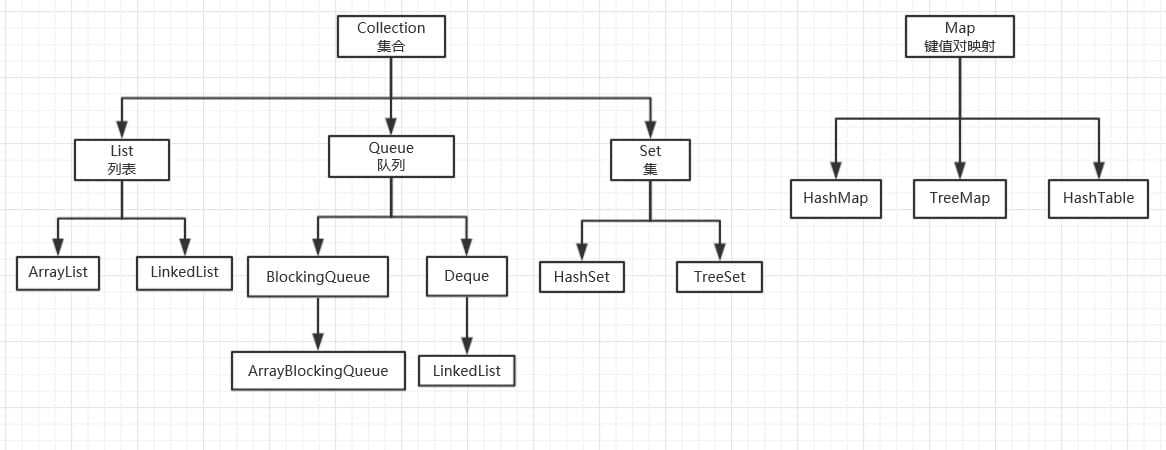### java迭代器的使用

        List<String> list = new ArrayList<>();

for (int i = 0; i < list.size(); i++){
if(list.get(i).equals("*")){
list.remove(i);
}
System.out.println(i + "|" + list.size());//注释代码
}
System.out.println(list);


0|3
1|2
[*, *]

        for (Iterator iterator = list.iterator(); iterator.hasNext();) {
String string = (String) iterator.next();
if(string.equals("*")){
iterator.remove();
}
}

### Iterater

hasNext();//如果迭代器指向位置后面还有元素，则返回 true，否则返回false

next();//返回集合中Iterator指向位置后面的元素

remove();//删除集合中Iterator指向位置后面的元素

### ListIterator

Iterator可以应用于所有的集合，Set、List和Map和这些集合的子类型。而ListIterator只能用于List及其子类型。

ListIterator多了很多有用的方法：

//获取当前元素索引（通过上一个或者下一个元素的索引间接获取）：
nextIndex()-1;
previousIndex()+1;

//倒序输出，获取ListIterator，要传递list大小作为参数：
for (ListIterator iterator = list.listIterator(list.size()); iterator.hasPrevious();) {
String string = (String) iterator.previous();
System.out.print(string);
}

//添加元素

//修改元素
set(E e);

### List

// 建立链表
List<Integer> list = new ArrayList();

//增加数据

//删除
list.remove(int index);
list.remove(Object object);//需要重写类的equal方法
iterator.remove();

// 指定位置插入数据
list.set(pos,val);//会覆盖pos位置的值（修改元素值）

// 查询指定位置的值
list.get(pos);

// 判断某元素值是否存在
list.contains(val);//返回true false
list.indexof(val);//返回第一个匹配项的位置或者-1

// 数组转list
ArrayList<String> list = new ArrayList<String>(Arrays.asList(strArray)) ;

//合并数组

//list转数组
String[] sss = listStrings.toArray(new String[listStrings.size()]);

//list转String

//排序
Collections.sort(list);// 默认升序
Collections.sort(listCollections.reverseOrder();//降序排序
//自定义排序接口，返回1表示，o1和o2要进行左右交换移动，-1表示不需要移动。0表示两个值相等。

//去除重复元素
list转set再转list
list = new ArrayList<>(new LinkedHashSet<>(list));//这里用LinkedHashSet是因为会保证元素的添加顺序和之前的list一致

https://www.cnblogs.com/dolphin0520/p/3681042.html

### set

Java中Set真的是无序的吗？

set好处就是没有重复元素，构造方法上面也可以看出来，可以直接把list转成set

### PriorityQueue

java优先队列，每次peek()总是输出一个按照指定规则的的元素（默认是取出最小的）。但是队列内部，并不是有序的。

//实现大顶堆，构建的时候传递Comparator参数
//初始化堆大小随便的，即使是1，也可以不断的添加新元素
Queue<ListNode> priorityQueue = new PriorityQueue<ListNode>([初始化堆大小],new Comparator<ListNode>(){

@Override
public int compare(ListNode o1, ListNode o2) {
return o1.val-o2.val;
}

});
//定义
PriorityQueue<Integer> list = new PriorityQueue<Integer>();

//增加元素
list.offer(int);

//获取队首元素，但不删除
list.peek();

//获取队首元素，且删除
list.poll();

## 栈Stack

Stack 继承自 Vector(动态数组，很多方法和list差不多)

Stack<Interger> stack = new Stack();
stack.isEmpty();//判断栈是否为空
stack.ClearStack：清空栈

stack.size();//即栈的长度

stack.peek();//返回栈顶元素

stack.push();//进栈

stack.pop();//返回栈顶元素，并删除栈顶元素

## Map

Map并不是简单的二元组，有一个特点是Key不能重复的。

//建立map
Map<Integer,Integer> map = new HashMap<>();

//插入/修改元素
map.put(key,val);
map.putAll(Map t)

// 按照Key 查询
map.get(key);

//删除元素
map.remove(key);

// 按key 排序
（降序排序）

//  map 转换成 list
Set<Map.Entry<Integer, Integer>> entrys =   map.entrySet();//利用这个获得map的键值对映射的集合

List<Map.Entry<Integer, Integer>> = new ArrayList<>(entrys);//list的元素类型就是set<Map.entry<Integer,Integer>> 类型

## 数组

//定义一个空数组
int [] empty = new int;
//定义一个有初值的数组
int [] have = new int[]{1,2,3};

## Math 类的常用方法


//计算a的b次幂，a^b
Math.pow(double a, double b)
System.out.println(Math.pow(2, 3)); // 8.0

//计算自然常数的a次幂，e^a
Math.exp(double a)
System.out.println(Math.exp(1)); // 2.718281828459045

//计算平方根，√a
Math.sqrt(double a)
System.out.println(Math.sqrt(16)); // 4.0

//计算立方根，a‾√3a3
Math.cbrt(double a)
System.out.println(Math.cbrt(8)); // 2.0

//计算以e为底的对数（自然对数），logexlog⁡ex 或 lnxln⁡x
Math.log(double x)
System.out.println(Math.log(Math.E)); // 1.0

//计算以10为底的对数，log10xlog⁡10x 或 lgxlg⁡x
Math.log10(double x)
System.out.println(Math.log10(100)); // 2.0

//计算绝对值，参数类型：int、long、float、double
Math.abs(int x)
System.out.println(Math.abs(-1)); // 1

//内部调用了 Random.nextDouble() 方法，生成一个伪均匀分布在0.0到1.0之间的double值
Math.random();
System.out.println((int)(Math.random() * 10 + 1)); // 生成范围再[1, 11)之间的伪随机数

//获取两个参数中的最大值，参数类型：int、long、float、double
Math.max(int x, int y)
System.out.println(Math.max(1, 2)); // 2

//获取两个参数中的最小值，参数类型：int、long、float、double
Math.min(int x, int y)
System.out.println(Math.min(1, 2)); // 1


## 正则表达式

• Patten 接收正则表达式作为参数
• MatcherMatcher基础上接收字符串作为参数

### 分割字符串

//方式1,用String类提供的方法
testString.split([正则表达式],[limit]);

//方式2
Patten p = Pattern.compile("\\d+");
p.split([string],[limit])
/* limit 选填，limit参数控制应用模式的次数，从而影响结果数组的长度。
1. 如果 n 大于零，那么模式至多应用 n- 1 次，数组的长度不大于 n，并且数组的最后条目将包含除最后的匹配定界符之外的所有输入。
2. 如果 n 非正，那么将应用模式的次数不受限制，并且数组可以为任意长度。
3. 如果 n 为零，那么应用模式的次数不受限制，数组可以为任意长度，并且将丢弃尾部空字符串。
*/

### 判断某段在字符串中是否存在以及查找匹配子串的位置

//方式1：Patten 静态方法或者实例方法【完全匹配】
Patten.matcher(String regex,CharSequence input);//返回 true or false
Patten.matches("\\d+","2223");//返回true
Patten.matches("\\d+","2223aa");//返回false
Patten.matches("\\d+","22bb23");//返回false

//方式2：Matcher 类方法
m.matches();//【完全匹配】，和上面效果一样

m.lookingAt();//【部分匹配】。223aa 返回true

//注意:通过m.matches()/m.lookingAt()/m.find() 都会移动指针到下一个匹配的字符串位置，会影响后续的m.find()操作的结果。
m.reset();//重置匹配指针的位置

Matcher m = p.mather(testString);
m.find();//【部分匹配】，返回true or false，而且指针会移动下次匹配的位置
while(m.find()) {
System.out.println("位置1" + m.start());
System.out.println("位置2" + m.end());
System.out.println(m.group());// m.group()返回当前匹配的字符串
System.out.println(m.groupCount());//返回当前匹配的组的数目
System.out.println(m.group(0));//返回当前字符串的第一组的字符串
}

https://blog.csdn.net/zengxiantao1994/article/details/77803960

## ScriptEngineManager

    ScriptEngineManager manager = new       ScriptEngineManager();
ScriptEngine engine = manager.getEngineByName("javascript");
//根据“脚本语言名称”获取执行引擎，java本身默认只支持javascript
//getEngineByName方法每次都会创建一个新的Engine对象
String script = "3+4*5";
int result = engine.eval(script);
System.out.println(total);  

https://shift-alt-ctrl.iteye.com/blog/1896690

## 数据结构和算法

### 数据结构

#### 树

https://www.ihewro.com/archives/895/#ProblemC 这一题中就用到了这个结构。

edges[child] = parent每个位置的值为该编号节点的父节点。

public class Tree {
public static void main(String[] args) {
Scanner scanner = new Scanner(System.in);

int n = scanner.nextInt();
//n个节点，n-1条边的信息
int [] edges = new int [n+1];//节点编号从1开始
edges = -1;
for (int i = 0; i < n-1; i++) {
int parent = scanner.nextInt();
int child = scanner.nextInt();
edges[child] = parent;

}
for (int i = 0; i < edges.length; i++) {
System.out.print(edges[i] + " | ");
}
System.out.println();
tree_print(edges);
}

public static void tree_print(int[] edges) {
for (int i = 1; i < edges.length; i++) {
System.out.println(i);
for (int j = 1; j < edges.length; j++) {
if (edges[j] == i) {
System.out.print(j + "|");
}
}
System.out.println();
}
}

}


#### 二叉树

https://www.ihewro.com/archives/904/#二叉排序树 这道题目用到这个树的结构，构建树，并且要求前中后遍历打印出来

    class TreeNode{
int value;
TreeNode left;
TreeNode right;

public TreeNode(int value) {
this.value = value;
this.left = null;
this.right = null;
}

public TreeNode() {
}
}

#### 图

https://www.ihewro.com/archives/894/#ProblemD.网络传输

    class MGraph {
int vertexNum;//顶点数目
int edgesNum;//边的数目
int[][] edges;//二维矩阵，表示任意两个点之间是否有边
}

### 算法

#### 迪杰斯特拉最短路径

https://www.ihewro.com/archives/894/#ProblemD

• 集合T，初始状态是除了顶点O之外的所有顶点集合。
• 集合S，初始状态只有顶点O
• dist[]，源点到其他所有顶点的距离，初始状态下，初始状态下，如果顶点O与其他顶点直接相连，值就边的权值，如果不相连，值为∞（编程中定义一个很大的数字）
• path[]，源点到目标节点的最短路径的前一个节点编号，初始状态下，如果顶点O与其他顶点直接相连，值就是源点O的编号，如果不相连，值为-1

• 每次从T集合吸一个节点到S集合中（吸星大法），这个节点的特征是源点O到该节点的路径长度最小
• 并使用这个节点作为中间者，修改源点到T集合其他节点（这里只要找T集合的其他节点即可，而不是除源点外的所有节点！！）的最短路径。如果dist[中间点] + 距离△ < dist[其他节点]：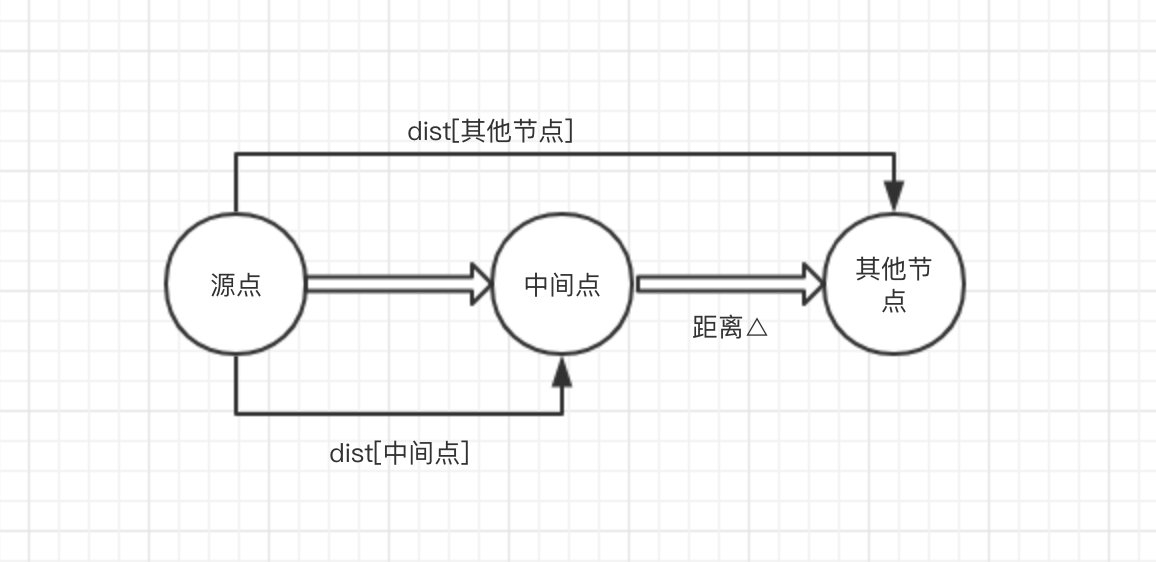* 修改dist[其他节点] = dist[中间点] + 距离△
* 修改path[其他节点] = 中间点
• 从T集合中移除该中间点

path = 4，只需要存储前一个节点编号就可以，而不需要存储这一串编号。这个思想其实在我们编程中也可以用到。

path = 4
path = 5
path =2
path = 1
path = 0

java 正无穷表示：通过Double或Float的POSITIVE_INFINITY表示。

T集合我用java的list 结构。

   class MGraph {
int vertexNum;
int edgesNum;
int[][] edges;

int[][] dist;
List<Integer> T = new ArrayList<Integer>();//T集合

public void initEmptyDist() {
dist = new int[vertexNum + 1][vertexNum + 1];
edges = new int[vertexNum + 1][vertexNum + 1];

for (int i = 1; i <= vertexNum; i++) {
for (int j = 1; j <= vertexNum; j++) {
dist[i][j] = -1;
edges[i][j] = -1;
}
}
}

public void Dijkstra(int origin) {

// 1.初始化T集合
for (int i = 1; i <= vertexNum; i++) {
if (i != origin) {
}
}

while (T.size() != 0) {
int selectVertex = -1;//源点到T集合中顶点最短的路径的顶点编号
int selectVertexInT = 0;//源点到T集合中顶点最短的路径的顶点在T集合中序号，因为后面要从T集合中删掉
int min = -1;//源点到T集合中顶点最短的路径

//2. 选择中间节点
for (int i = 0; i < T.size(); i++) {
if (dist[origin][T.get(i)] != -1 && (dist[origin][T.get(i)] < min || min == -1)) {
min = dist[origin][T.get(i)];
selectVertex = T.get(i);
selectVertexInT = i;
}
}

// 3. 使用中间点修改T集合中其他节点
for (int i = 0; i < T.size(); i++) {
int currentTargetVertex = T.get(i);
int query = query(selectVertex, currentTargetVertex);
if (query != -1) {
int modified = min + query(selectVertex, currentTargetVertex);
if (dist[origin][currentTargetVertex] == -1 || dist[origin][currentTargetVertex] > modified) {
dist[origin][currentTargetVertex] = modified;
}
}
}

//4. 从T集合中删除中间节点
T.remove(selectVertexInT);
}
}

//查询图中两个顶点间的距离
public int query(int start, int end) {
int result = -1;
if (edges[start][end]!= -1) {
result = edges[start][end];
}
return result;
}
}


#### floyd 最短路径

https://www.ihewro.com/archives/893/#ProblemC

（效果等价于对每个节点使用迪杰斯特拉算法，但是弗洛伊德算法的时间复杂度很高o(n³)）

• dist[][]，任意两个节点的最短路径的值，初始状态就是图中两个节点边的值，初始状态就是临界矩阵
• path[][]，任意两个节点的最短路径的前一个节点，初始状态所有值为-1，表示没有中间点（这个初始值和迪杰斯特拉不一样）

• 把每个顶点作为中间顶点，然后比较disti 与 disti+distk 判断是否修改最短路径。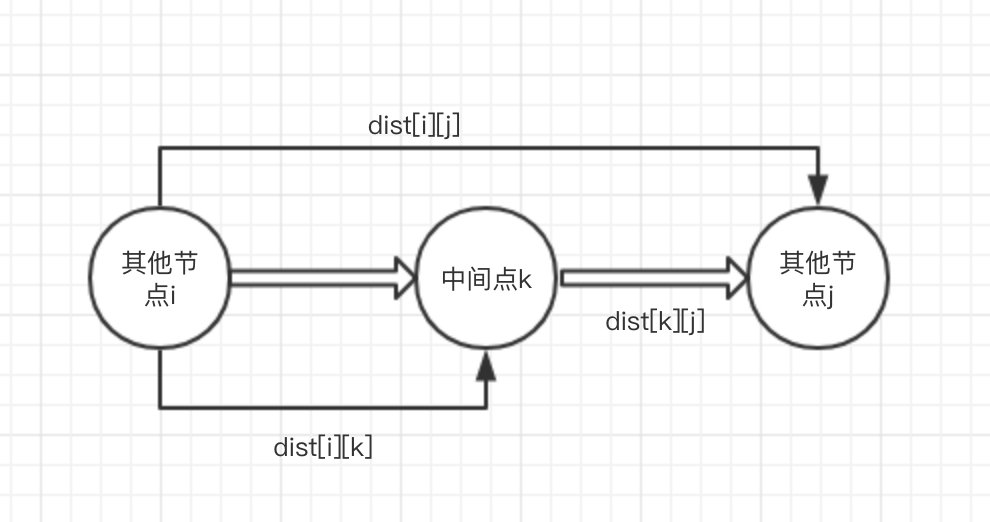MGraph mGraph = new MGraph();
//给图赋值代码省略
//1.初始化dist[][]数组
mGraph.initA_array();

//2. 三重循环，把每个顶点作为中间点
for (int i = 1;i<= mGraph.getVertexNum(); i++){// circle each vertex as an intermediate point
for (int k=1;k <= mGraph.getVertexNum();k++){
for (int j = 1;j <= mGraph.getVertexNum();j++){
if (mGraph.A[i][j] > mGraph.A[i][k] + mGraph.A[k][j]){
mGraph.A[i][j] = mGraph.A[i][k] + mGraph.A[k][j];
}
}
}
}

class MGraph{
int vertexNum;
int edgeNum;

int edges[][] = new int;
int A[][] = new int;//就是dist[][]

//实例化图的时候，需要先将任意两个节点的边赋值为-1
public MGraph() {
for (int i = 0;i<51;i++){
for (int j = 0; j< 51;j++){
edges[i][j] = -1;//init edges array
}
}
}

//初始化dist数组，等价于edge[][]邻接矩阵数组
public void initA_array(){
for (int i = 1;i<=this.getVertexNum();i++){
for (int j = 1;j<=this.getVertexNum();j++){
if (edges[i][j] == -1){//两个节点没有边
A[i][j] = this.vertexNum;//把节点数作为不可达，因为两个几点的距离不可能超过节点数的大小
}else {
A[i][j] = edges[i][j];
}
}
}
}
}

#### 全排列

c++内置全排列算法，羡慕。

        permutation(list, 0, list.size() - 1);

public void permutation(List<Integer> list, int from, int to) {
if (to < from)
return;
if (from == to) {
//此时list是一个新的排列

} else {
for (int i = from; i <= to; i++) {
swap(list, i, from);
permutation(list, from + 1, to);
swap(list, from, i);
}
}
}

public void swap(List<Integer> s, int i, int j) {
int tmp = s.get(i);
s.set(i, s.get(j));
s.set(j, tmp);
}

## eclipse 设置

### 代码提示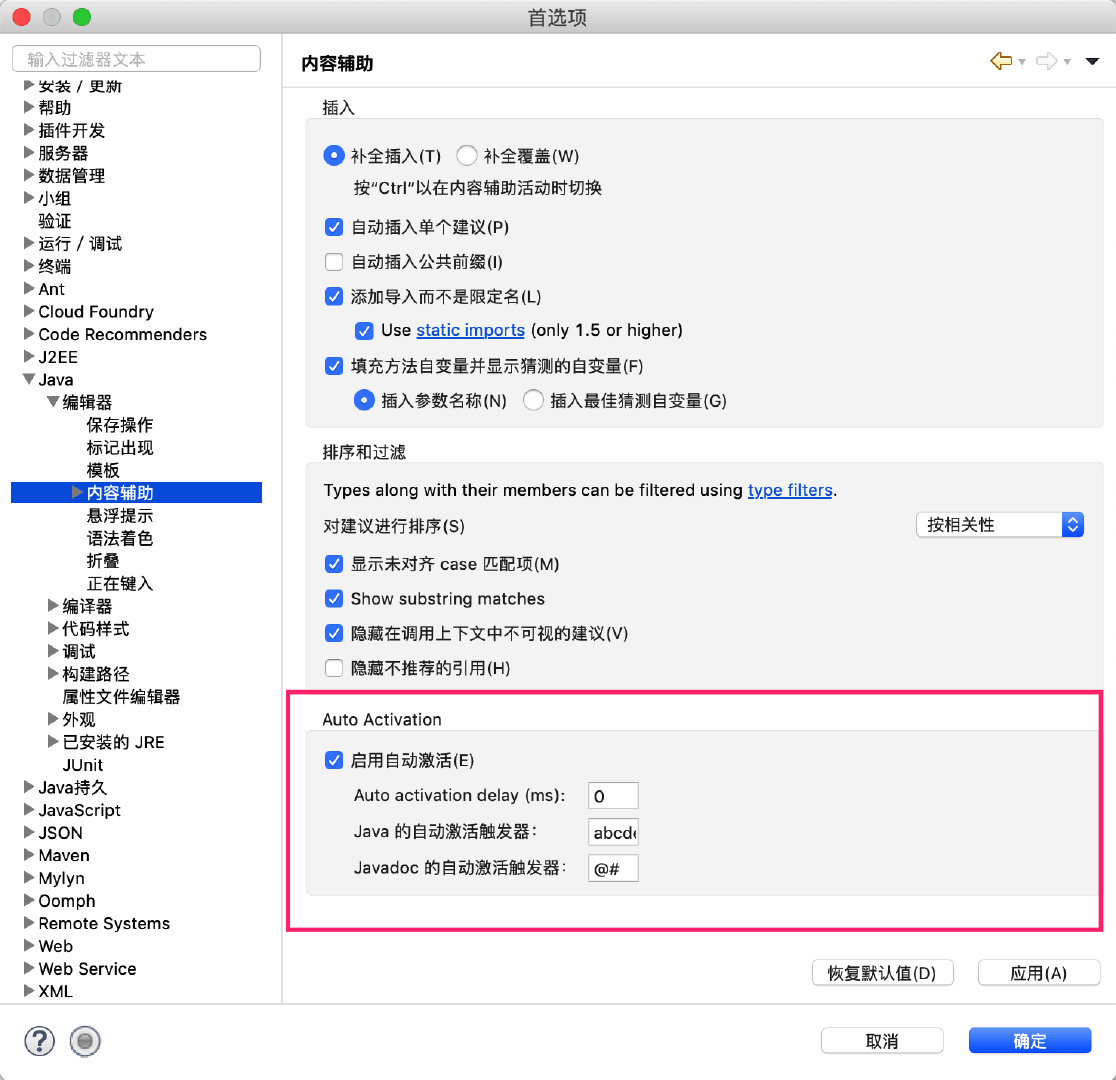把.修改成.abcdefghijklmnopqrstuvwxyzABCDEFGHIJKLMNOPQRSTUVWXYZ(,

https://blog.csdn.net/ithomer/article/details/7090405

### eclipse 常用快捷键

// command = ctrl (windows)

Alt + / 提示
command + / 当行注释
command + d 删除当前行
command + 1  快速修复
syso :     System.out.println();

## OJ常见问题

### 样例正确，AC失败

1. 特殊样例没有考虑到，比如2014网研分数加法第一题
2. 复制过去代码不要有包名 package bupt;他不提示编译错误，但是答案就是错误...
3. 首先确定自己是否正确理解题意
4. 然后测试临界条件，特殊条件
5. 运行时错误：内存超限，并不是某个数组或集合取数超限，而是内存超限

### 一些注意的地方

1. 变量初始化位置要注意，如果写在循环内部和循环外部就差远了
2. 注释在AC成功之前不要删掉，否则后面还得重新加注释……

### == 和 equals 使用区别

• 对于==如果作用于基本数据类型的变量，则直接比较其存储的 “值”是否相等；如果作用于引用类型的变量，则比较的是所指向的对象的地址
• 对于equals方法
如果没有对equals方法进行重写，则比较的是引用类型的变量所指向的对象的地址；
诸如String、Date等类默认对equals方法进行了重写，比较的是所指向的对象的内容。
注意：equals方法不能作用于基本数据类型的变量。

http://www.cnblogs.com/dolphin0520/p/3592500.html

### 北邮OJ上机注意点

1. 北邮支持c/c++/java7 （我选的是java）
2. java 提交代码时候，记得删掉包名，类名称改为Main,入口函数为main(String[] args);
3. 提交代码的界面：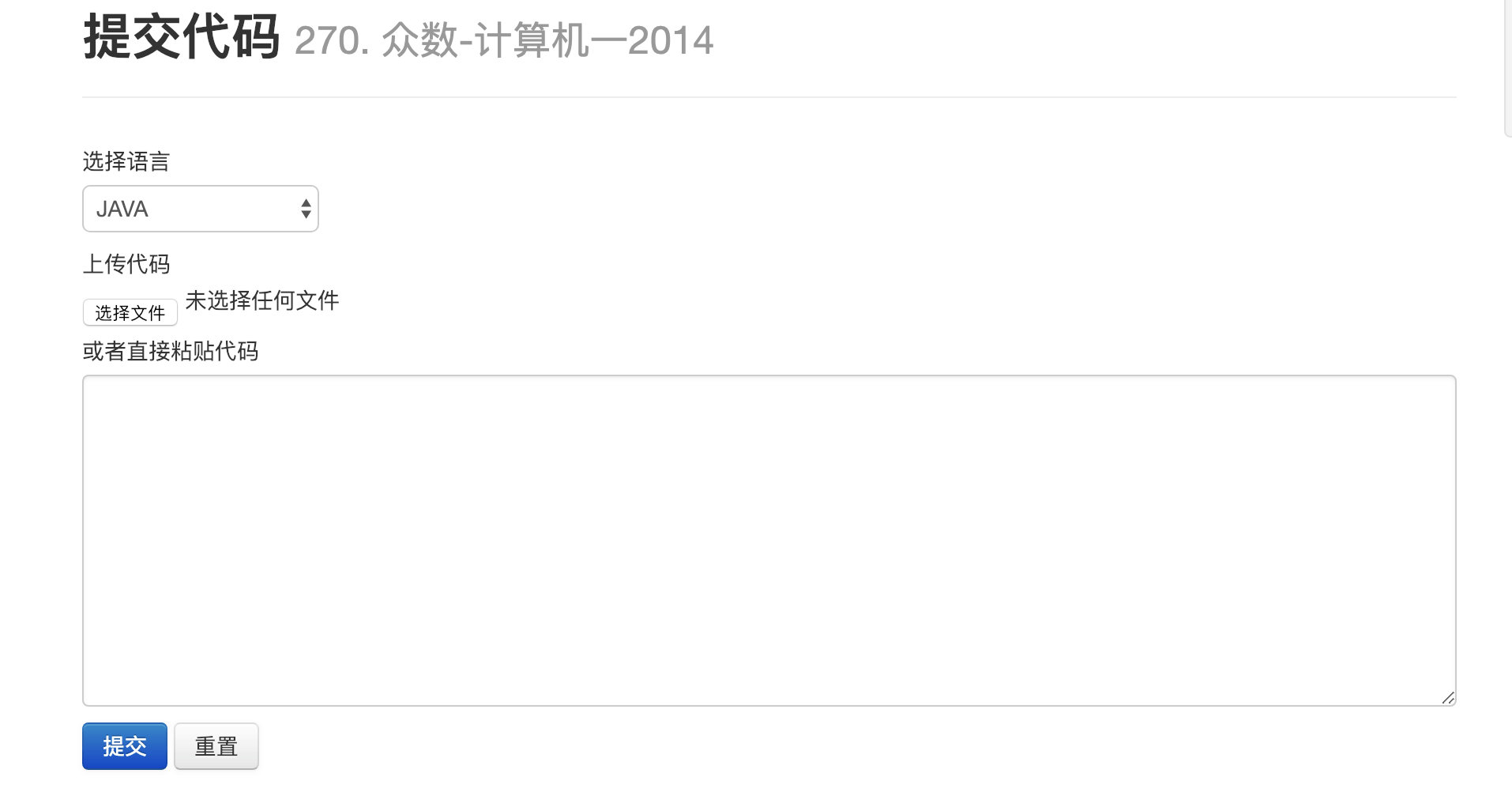4. 检查结果的界面： （答案错误，没有任何提示！）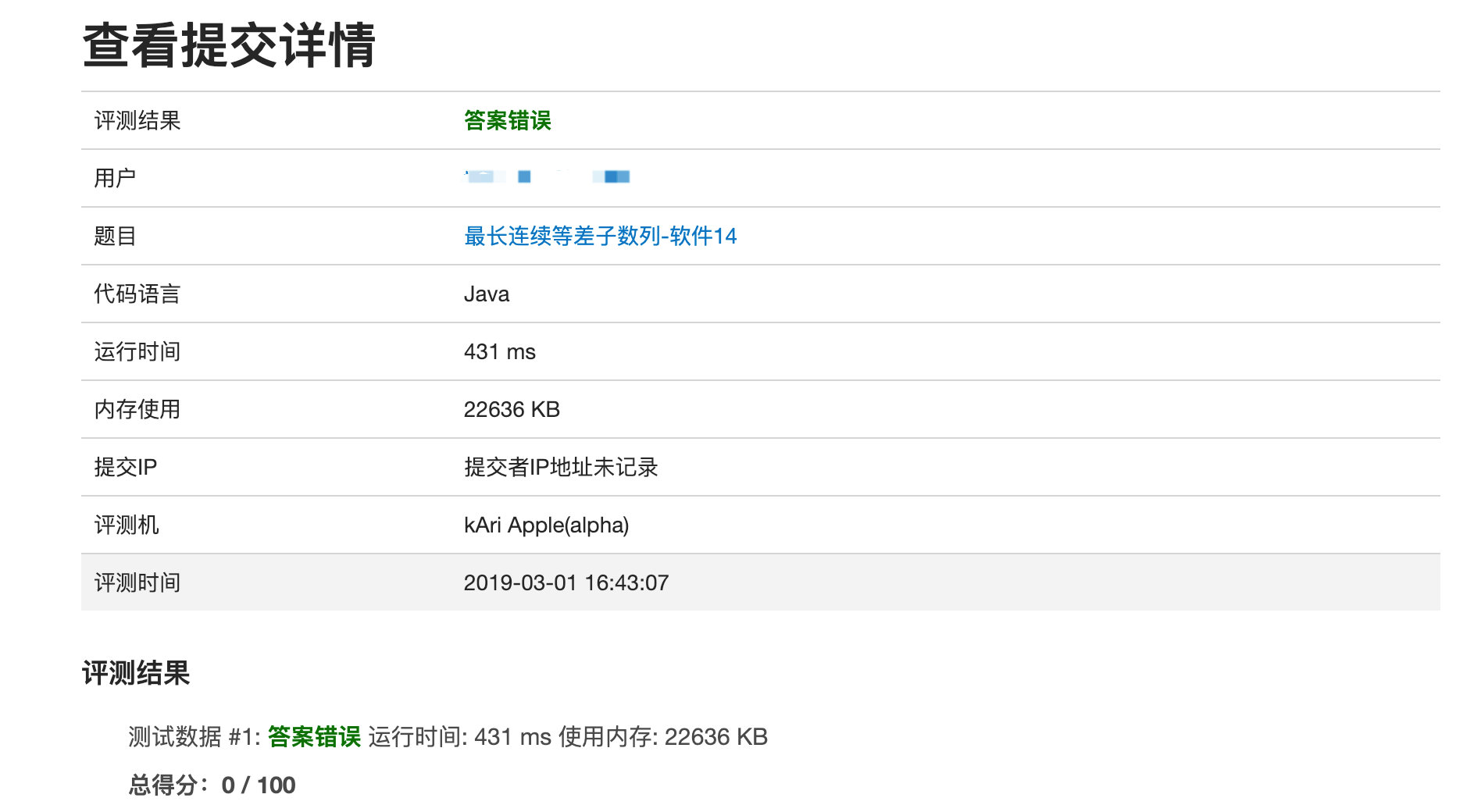5. 不要有中文注释，不支持中文编码